Untitled83

# IMAGE SIMILARITY using Siamese Network with Triplet Loss�

A Siamese networks consists of two identical neural networks, each taking one of the two input images. The last layers of the two networks are then fed to a contrastive loss function , which calculates the similarity between the two images.Each image in the image pair is fed to one of these networks.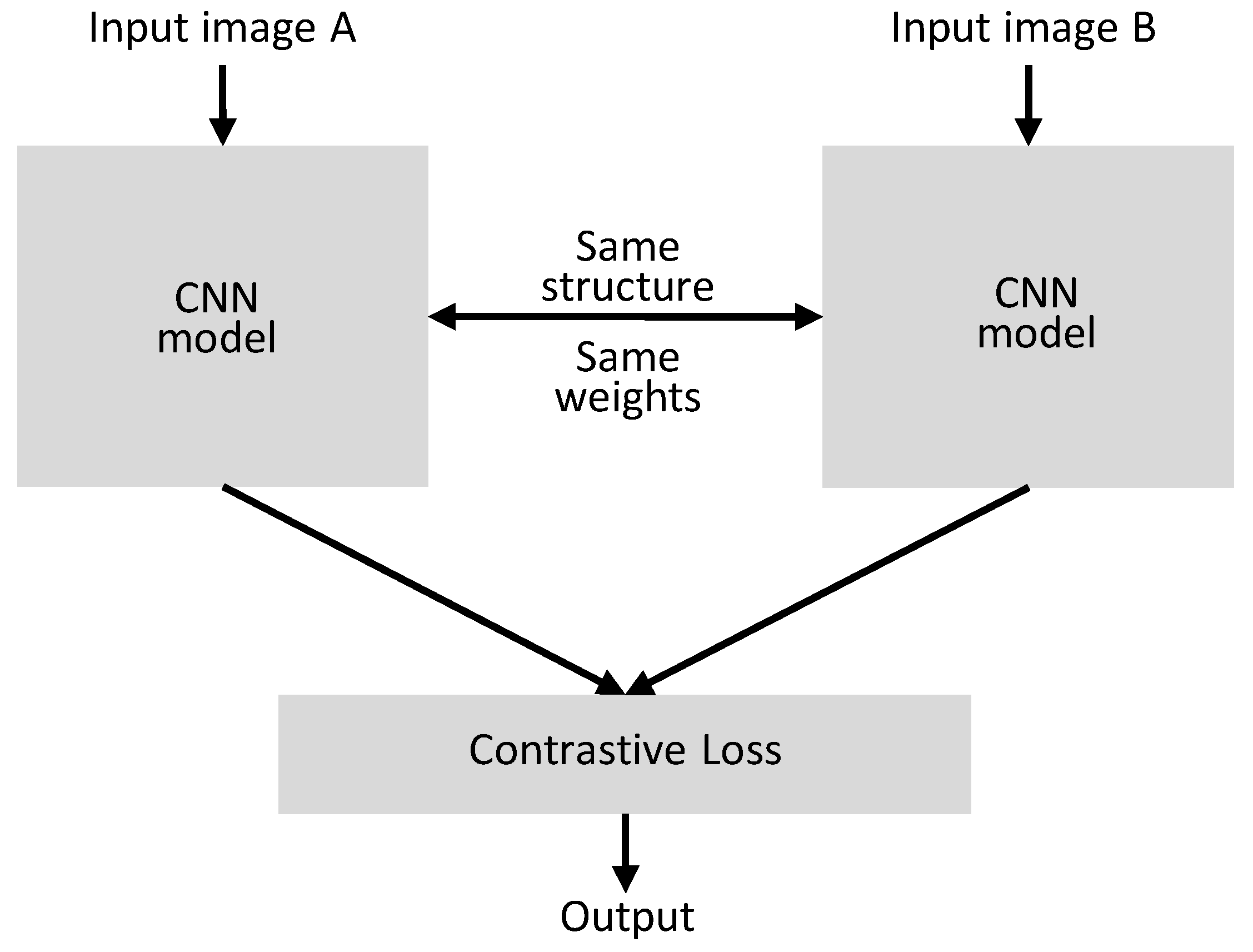Triplet loss is a loss function for machine learning algorithms where a baseline (anchor) input is compared to a positive (truthy) input and a negative (falsy) input.This can be avoided by posing the problem as a similarity learning problem instead of a classification problem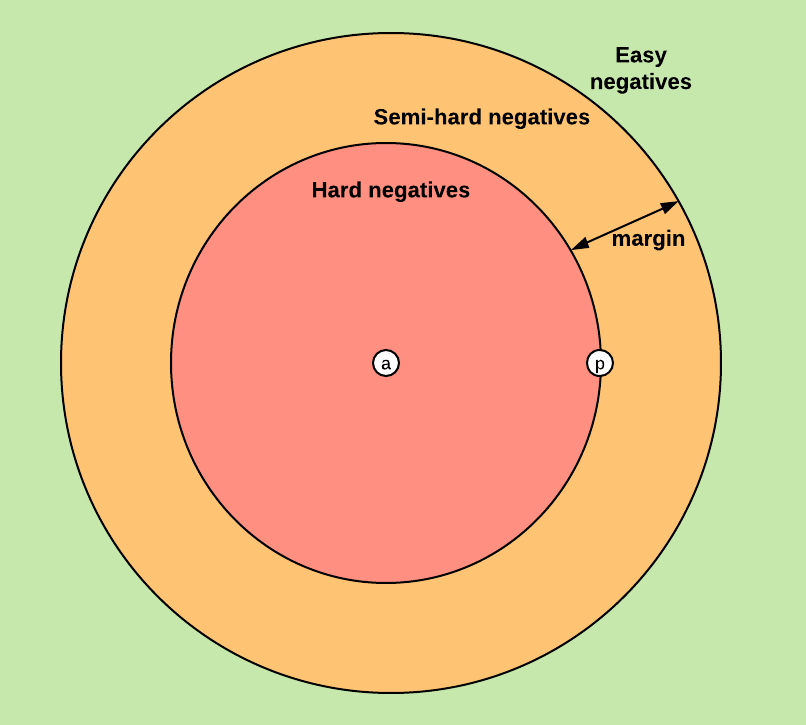In :
# loading libraries
import random
import numpy as np
import tensorflow as tf
import matplotlib.pyplot as plt
from tensorflow.keras.datasets import mnist

from keras.models import Sequential
from keras.layers import Conv2D, ZeroPadding2D, Activation, Input, concatenate, Dropout, GlobalAveragePooling2D
from keras.models import Model
from keras.layers.pooling import MaxPooling2D
from keras.layers.merge import Concatenate
from keras.layers.core import Lambda, Flatten, Dense
from keras.initializers import glorot_uniform
from sklearn.preprocessing import LabelBinarizer
from keras.optimizers import *
from keras.engine.topology import Layer
from keras import backend as K
from keras.regularizers import l2
from keras.utils.vis_utils import plot_model

In :
#loading MNIST dataset
(x_train, y_train), (x_test, y_test) = mnist.load_data()

print(f'Training data shape: {x_train.shape}, Testing data shape: {x_test.shape}')

Training data shape: (60000, 28, 28), Testing data shape: (10000, 28, 28)

In :
# normalizing images
x_train = x_train / 255.
x_test  = x_test / 255.

print(f'Training data shape: {x_train.shape}, Testing data shape: {x_test.shape}')

Training data shape: (60000, 28, 28), Testing data shape: (10000, 28, 28)

In :
#output embedding dimension
embeddingDim=48

# Siamese network
embedding_model = tf.keras.models.Sequential([

Conv2D(32, kernel_size = 3, activation='relu', input_shape = (28, 28, 1)),
Conv2D(32, kernel_size = 3, activation='relu'),
Conv2D(32, kernel_size = 5, strides=2, padding='same', activation='relu'),

Conv2D(64, kernel_size = 3, activation='relu'),
Conv2D(64, kernel_size = 3, activation='relu'),
Conv2D(64, kernel_size = 5, strides=2, padding='same', activation='relu'),
Conv2D(128, kernel_size = 4, activation='relu'),

# prepare the final outputs
GlobalAveragePooling2D(),
Dense(embeddingDim)
])

In :
#input layer for anchor image
input_anchor = tf.keras.layers.Input(shape = (28, 28, 1))

#input layer for positive image
input_positive = tf.keras.layers.Input(shape = (28, 28, 1))

#input layer for negative image
input_negative = tf.keras.layers.Input(shape = (28, 28, 1))

In :
embedding_anchor = embedding_model(input_anchor)
embedding_positive = embedding_model(input_positive)
embedding_negative = embedding_model(input_negative)

In :
#concatenating output of each input
output = tf.keras.layers.concatenate([embedding_anchor, embedding_positive, embedding_negative], axis = 1)

#final model
model = tf.keras.models.Model([input_anchor, input_positive, input_negative], output)

#plotting model architecture
plot_model(model, to_file='model_plot.png', show_shapes=True, show_layer_names=True)

Out: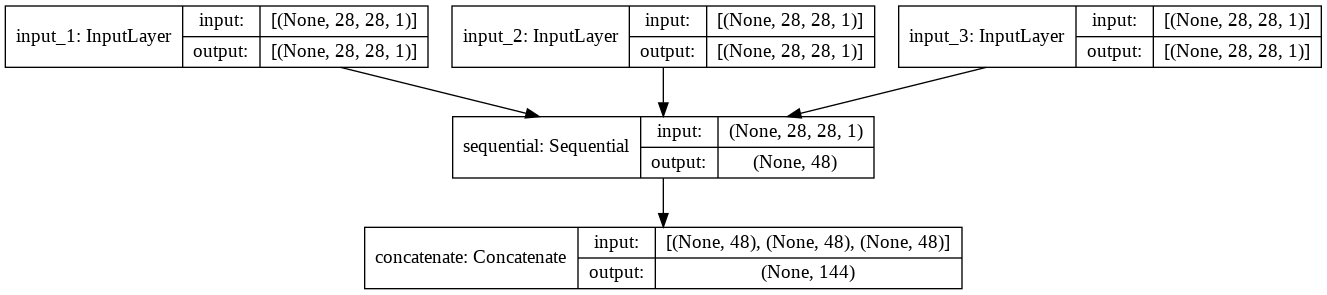In :
# increasing dimension of input images across third axis
x_train = np.expand_dims(x_train, axis=3)
x_test = np.expand_dims(x_test, axis=3)

print(f'Training data shape: {x_train.shape}, Testing data shape: {x_test.shape}')

Training data shape: (60000, 28, 28, 1), Testing data shape: (10000, 28, 28, 1)

In :
# it will create image dataset for anchor, positive and negative images

def create_batch_data(batch_size = 256):

# all of these numpy arrays are of same size
anchors,positives,negatives   = np.zeros((batch_size, 28, 28, 1)), np.zeros((batch_size, 28, 28, 1)), np.zeros((batch_size, 28, 28, 1))

for i in range(0, batch_size):
# generating random number between 0 and 60000(total number of images)
index = random.randint(0, x_train.shape - 1)

# indexe for anchor image and it's label
anchor   = x_train[index]
y     = y_train[index]

# list of positive label indexes
index_pos = np.where(y_train == y)
index_pos = np.squeeze(index_pos)

# list of negative label indexes
index_neg = np.where(y_train != y)
index_neg = np.squeeze(index_neg)

# length of positive list containing indexes
len_pos = len(index_pos)

# length of negative list containing indexes
len_neg = len(index_neg)

# randomly selecting any single image from all the list of indexes
positive = x_train[index_pos[random.randint(0, len_pos - 1)]]
negative = x_train[index_neg[random.randint(0, len_neg - 1)]]

anchors[i], positives[i], negatives[i]   = anchor, positive, negative

return [anchors, positives, negatives]

In :
# triplet loss
def Triplet_loss(alpha, embedding_dim):
def get_loss(y_true, y_pred):

# getting output embedding dimension of positive, negative and anchor images
anchor_output = y_pred[:, : embedding_dim]
positive_output = y_pred[:, embedding_dim : 2*embedding_dim]
negative_output = y_pred[:, 2*embedding_dim:]

dp = tf.reduce_mean(tf.square(anchor_output - positive_output), axis = 1)
dn = tf.reduce_mean(tf.square(anchor_output - negative_output), axis = 1)

return tf.maximum(dp - dn + alpha, 0.0)
return get_loss

In :
def Data_generator(batch_size, embedding_dim):
while True:
# getting input image and it's label
x = create_batch_data(batch_size)
y = np.zeros((batch_size, 3*embedding_dim))

yield x, y

In :
batch_size = 1
epochs = 5
embedding_dim = 48
model.compile(loss = Triplet_loss(alpha = 0.4, embedding_dim = embedding_dim), optimizer = 'adam')

In :
# training over dataset
history = model.fit(Data_generator(batch_size, embeddingDim),
steps_per_epoch = int(x_train.shape/batch_size),
epochs = epochs)

Epoch 1/5
60000/60000 [==============================] - 1370s 23ms/step - loss: 0.1053
Epoch 2/5
60000/60000 [==============================] - 1426s 24ms/step - loss: 0.0259
Epoch 3/5
60000/60000 [==============================] - 1437s 24ms/step - loss: 0.0209
Epoch 4/5
60000/60000 [==============================] - 1434s 24ms/step - loss: 0.0207
Epoch 5/5
60000/60000 [==============================] - 1445s 24ms/step - loss: 0.0185

In :
# summarize history for loss
plt.plot(history.history['loss'])

plt.ylabel('loss')
plt.xlabel('epoch')

plt.legend(['train_loss'], loc='upper right')
plt.show()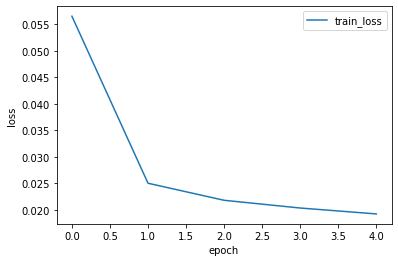In :
#calculating cosine similarity between two images.

def cosine_similarity(a, b):

nominator = np.dot(a, b)
a_norm = np.sqrt(np.sum(a**2))
b_norm = np.sqrt(np.sum(b**2))

denominator = a_norm * b_norm
cosine_similarity = nominator / denominator

return cosine_similarity

In :
#calculating similarity between three images given below
plt.figure(figsize=(2,2))

# negative image
plt.subplots(1,1)
plt.axis('off')
plt.imshow(np.squeeze(x_test, axis=2))
plt.title('negative')

#positive image
plt.subplots(1,1)
plt.axis('off')
plt.imshow(np.squeeze(x_test, axis=2))
plt.title('positive')

#anchor image
plt.subplots(1,1)
plt.axis('off')
plt.imshow(np.squeeze(x_test, axis=2))
plt.title('anchor')

Out:
Text(0.5, 1.0, 'anchor')
<Figure size 144x144 with 0 Axes>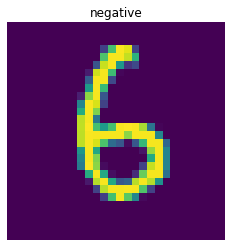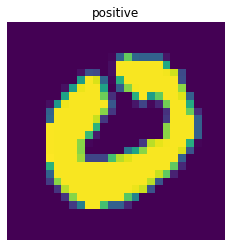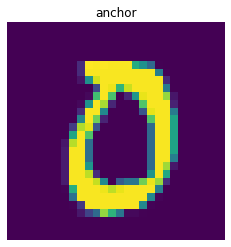In :
negative_img = x_test.reshape(-1, 28, 28, 1)
positive_img = x_test.reshape(-1, 28, 28, 1)
anchor_img = x_test.reshape(-1, 28, 28, 1)

prediction = model.predict([anchor_img, positive_img, negative_img])


Similarity between positive and anchor image

In :
print("similarity-score between positive and anchor image: ", cosine_similarity(prediction[:48], prediction[48:96]))

similarity-score between positive and anchor image:  0.9425622


Similarity between anchor and negative image

In :
print("similarity-score between anchor and negative image: ", cosine_similarity(prediction[:48], prediction[96:]))

similarity-score between anchor and negative image:  0.2961074


From the above result I conclude that positive and anchor images are similar to each other whereas anchor and negative images are dissimilar to each other.#### More Articles of ML_coderzz unknown:

Name Views Likes
Facial Attribute Analysis 509 1
Generate QR Codes with Python and add Style 434 1
Create a simple chatbot in Python 226 1
Currency Converter using python 208 1
Pixel Level Image Comparison 1752 1
Neural Style Transfer With 2 Lines Of Code 341 1
Graph Based Text Representation 274 1
Semantic Segmentation With 2 Lines Of Code 336 1
Pose Estimation With 4 Lines Of Code 300 1
Question the Text and Summarize it 408 1
Cartooning an Image 247 1
Image to Sketch 245 1
Fine Tuning BERT for Text classification 569 1
Encoding-Decoding Text-to-Image 758 1
Create Pattern in Python 195 1
Spell Checker 419 1
Cropping Part of an image using OpenCV (grabcut algorithm) 1253 2
Transformers : All In One 449 1
Deep Convolutional GAN (DCGAN) 515 1
Spectral Normalization for Generative Adversarial Networks (SN-GAN) 712 1
ENGLISH DICTIONARY using PYTHON 357 2
GENDER CLASSIFICATION 590 1
India Air Quality Data Analysis 526 1
WINE QUALITY PREDICTION 1456 1
PREDICT NEXT N WORDS TO GENERATE COMPLETE SENTENCE 409 2
Denoising colored image 360 1
Print Emojis in Python 256 0
EMOTION DETECTION FROM TEXT 407 2
WORD EMBEDDING REPRESENTATION AND VISUALISATION (using GLOVE vector) 1016 2
Age Detection of Actors 1067 2
IMAGE CLASSIFICATION USING PRE-TRAINED MODEL 611 1
MNIST HANDWRITTEN DIGIT CLASSIFICATION (end to end project using TensorFlowJS) 667 2
IMAGE SIMILARITY USING SIAMESE NETWORK WITH TRIPLET LOSS 3199 1
CONDITIONAL GAN 1020 3
SIMILAR SENTENCE GENERATION 1532 1
TEXT TO SPEECH using gTTS 385 2
PLANT DISEASE CLASSIFICATION (end to end project) 745 1
WASSERSTEIN GAN WITH GRADIENT PENALTY (WGAN GP) 909 1
TRAFFIC SIGN CLASSIFICATION 721 1
K-MEANS CLUSTERING OVER IMAGES 1073 1
Hindi OCR 984 1
GENERATING HANDWRITTEN MNIST DIGITS USING GAN 461 0
CURVE SIMILARITY MEASUREMENT 3048 2
Stock Price Prediction and Forecasting using LSTM 1267 1
LANGUAGE TRANSLATION 603 2
FACIAL EXPRESSION RECOGNITION 684 1
FACE COUNTING (COUNT THE NUMBER OF FACES PRESENT IN AN IMAGE) 2182 2
Image Quality Assessment 3098 1
Text Extraction From An Image 1128 2
NAMED ENTITY RECOGNITION 532 2

# IMAGE SIMILARITY using Siamese Network with Triplet Loss�

A Siamese networks consists of two identical neural networks, each taking one of the two input images. The last layers of the two networks are then fed to a contrastive loss function , which calculates the similarity between the two images.Each image in the image pair is fed to one of these networks.Triplet loss is a loss function for machine learning algorithms where a baseline (anchor) input is compared to a positive (truthy) input and a negative (falsy) input.This can be avoided by posing the problem as a similarity learning problem instead of a classification problemIn :

# loading librariesimport randomimport numpy as npimport tensorflow as tfimport matplotlib.pyplot as pltfrom tensorflow.keras.datasets import mnistfrom keras.models import Sequentialfrom keras.layers import Conv2D, ZeroPadding2D, Activation, Input, concatenate, Dropout, GlobalAveragePooling2Dfrom keras.models import Modelfrom keras.layers.pooling import MaxPooling2Dfrom keras.layers.merge import Concatenatefrom keras.layers.core import Lambda, Flatten, Densefrom keras.initializers import glorot_uniformfrom sklearn.preprocessing import LabelBinarizerfrom keras.optimizers import *from keras.engine.topology import Layerfrom keras import backend as Kfrom keras.regularizers import l2from keras.utils.vis_utils import plot_model

In :

#loading MNIST dataset(x_train, y_train), (x_test, y_test) = mnist.load_data()print(f'Training data shape: {x_train.shape}, Testing data shape: {x_test.shape}')

Training data shape: (60000, 28, 28), Testing data shape: (10000, 28, 28)

In :

# normalizing imagesx_train = x_train / 255.x_test  = x_test / 255.print(f'Training data shape: {x_train.shape}, Testing data shape: {x_test.shape}')

Training data shape: (60000, 28, 28), Testing data shape: (10000, 28, 28)

In :

#output embedding dimensionembeddingDim=48# Siamese networkembedding_model = tf.keras.models.Sequential([      Conv2D(32, kernel_size = 3, activation='relu', input_shape = (28, 28, 1)),    Conv2D(32, kernel_size = 3, activation='relu'),    Conv2D(32, kernel_size = 5, strides=2, padding='same', activation='relu'),    Conv2D(64, kernel_size = 3, activation='relu'),    Conv2D(64, kernel_size = 3, activation='relu'),    Conv2D(64, kernel_size = 5, strides=2, padding='same', activation='relu'),    Conv2D(128, kernel_size = 4, activation='relu'),	  # prepare the final outputs	  GlobalAveragePooling2D(),	  Dense(embeddingDim)])

In :

#input layer for anchor imageinput_anchor = tf.keras.layers.Input(shape = (28, 28, 1))#input layer for positive imageinput_positive = tf.keras.layers.Input(shape = (28, 28, 1))#input layer for negative imageinput_negative = tf.keras.layers.Input(shape = (28, 28, 1))

In :

embedding_anchor = embedding_model(input_anchor)embedding_positive = embedding_model(input_positive)embedding_negative = embedding_model(input_negative)

In :

#concatenating output of each inputoutput = tf.keras.layers.concatenate([embedding_anchor, embedding_positive, embedding_negative], axis = 1)#final modelmodel = tf.keras.models.Model([input_anchor, input_positive, input_negative], output)#plotting model architectureplot_model(model, to_file='model_plot.png', show_shapes=True, show_layer_names=True)

Out: